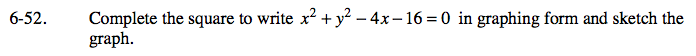### Home > CAAC > Chapter 13 > Lesson 13.IF3-S > Problem6-52

6-52.

Complete the square to write x2 + y2 − 4x −16 = 0 in graphing form and sketch the graph. Homework Help ✎(x2 − 4x + 4) − 4 + y2 − 16 = 0